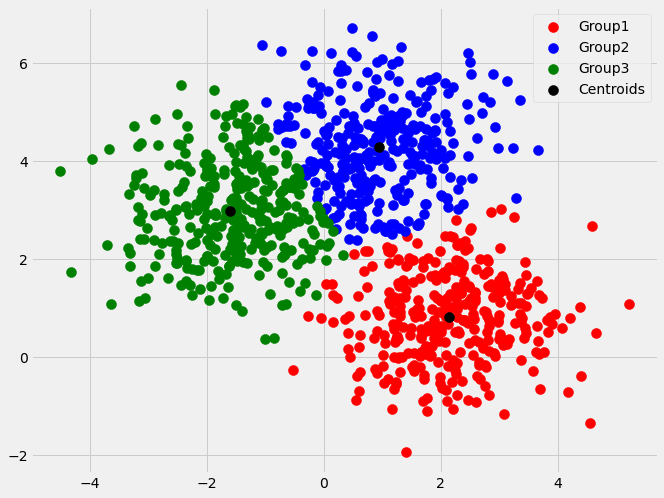# 3 minute read to ‘How to find optimal number of clusters using K-means Algorithm’Basically it is the sum of squared distance (usually Euclidean distance) from it's nearest centroid (center point of cluster). It decreases with increasing number of clusters(k). Aim is to find the bend (like an elbow joint) point in the graph.

Usually in any K-means clustering problem, the first problem that we face is to decide the number of clusters(or classes) based on the data. This problem can be resolved by 3 different metrics(or methods) that we use to decide the optimal ‘k’ cluster values. They are:

1. Elbow Curve Method
2. Silhouette Score
3. Davies Bouldin Index

Let us take a sample dataset and implement the above mentioned methods to understand their working.

We will use the make blobs dataset from sklearn.datasets library for illustrating the above methods

``````from sklearn.datasets import make_blobs

X, y = make_blobs(n_samples=1000, n_features=2,random_state=0)``````

Now let’s look at what these methods area and that after implementing those three methods on the created dataset what are the results.

## K-Means Clustering: How It Works & Finding The Optimum Number Of Clusters In The Data

K-Means Clustering: How It Works & Finding The Optimum Number Of Clusters In The Data. Mathematical formulation, Finding the optimum number of clusters and a working example in Python

## Basics of Machine Learning: K-Means Clustering

Basics of Machine Learning: K-Means Clustering. As we dive into the world of “Unsupervised” Machine Learning, we will encounter problems that would require us to cluster the data available to us.

## Most popular Data Science and Machine Learning courses — July 2020

Most popular Data Science and Machine Learning courses — August 2020. This list was last updated in August 2020 — and will be updated regularly so as to keep it relevant

## Difference between Machine Learning, Data Science, AI, Deep Learning, and Statistics

In this article, I clarify the various roles of the data scientist, and how data science compares and overlaps with related fields such as machine learning, deep learning, AI, statistics, IoT, operations research, and applied mathematics.

## 15 Machine Learning and Data Science Project Ideas with Datasets

Learning is a new fun in the field of Machine Learning and Data Science. In this article, we’ll be discussing 15 machine learning and data science projects.